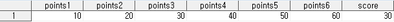data temp;
array points{3,2} (10,20,30,40,50,60);
score = points{2,1};
run;I don't understand why the variable names are point1-6 and the score is 30.

Please explain this array in detail because I'm unfamiliar with it.

Thank You.

7 REPLIES 7

## Re: About basic array question

@randomman wrote:

I don't understand why the variable names are point1-6

In SAS lingo, the variable names are point1-point6, not point1-6. When you don't specify variable names, the ARRAY assigns the variables to have the same name as the array name, but with suffixes indicating the position within the array. If you had specifically requested the array to use specific variable names, then you get those specific variable names, such as:

``````array points{3,2} flintstones jetsons scoobydoo bugsbunny elmerfudd roadrunner (10,20,30,40,50,60);
``````

I don't understand why ... the score is 30.

``````data temp;
array points{3,2} (10,20,30,40,50,60);
score1=points(1,1);
score2=points(1,2);
score3=points(2,1);
score4=points(2,2);
score5=points(3,1);
score6=points(3,2);
run;``````

--
Paige Miller

## Re: About basic array question

If you want to use different names than the automatic ones then include them in the ARRAY statement.

I find it help my brain to see the pattern if I use commas between the rows and spaces between the values within the rows when specifying initial values for a 2D array.  SAS does not care if you use spaces or commas (or some combination of the two) as the delimiters between such a list of values.

```224  data test;
225  array points{3,2} r1c1 r1c2 r2c1 r2c2 r3c1 r3c2
226    (10 20
227    ,30 40
228    ,50 60)
229  ;
230  put (_all_) (=/);
231  run;

r1c1=10
r1c2=20
r2c1=30
r2c2=40
r3c1=50
r3c2=60
NOTE: The data set WORK.TEST has 1 observations and 6 variables.
```

## Re: About basic array question

Is the question why the base of the names if POINTS?  What else would it make sense to use?

Is the question why are their six variables in the array?  That is because 3 times 2 is 6.

```232  data test;
233  array points[3,2] (1:6);
234  array scores (1:6);
235  put (_all_) (=/);
236  run;

points1=1
points2=2
points3=3
points4=4
points5=5
points6=6
scores1=1
scores2=2
scores3=3
scores4=4
scores5=5
scores6=6
```

## Re: About basic array question

array points{3,2} (10,20,30,40,50,60);

This statement

1. creates the variables points1-points6 as an enumeration of the array name points, because no individual varieble names are specified, and there are 6 values in the list.
2. asssigns the values 10-60 from the value list in the specified order,
3. and arranges the variables in a two-dimensional array structure {3,2} with 3 rows and 2 columns, where the variables are placed in row-column order.

So the result is:
|--------------|---------------|
| points1=10  | points2=20  |
|--------------|---------------|
| points3=30 | points4=40 |
|--------------|---------------|
| points5=50 | points6=60  |
|--------------|---------------|

score = points{2,1};

This statement picks the value from row 2 column 1 in the array structure, which is the value of the variable point3, = 30.
So score = points{2,1} returns the same value as score = points3.

## Re: About basic array question

Thanks @ErikLund_Jensen,

I understood it "arranges the variables in a two-dimensional array structure {3,2} with 3 rows and 2 columns, where the variables are placed in row-column order."

this part.

But, I didn't understand why the array looked like structure {1,6} not {3,2} in the result when I printed temp.

## Re: About basic array question

Except in PROC IML, a 3x2 rectangular structure of data does not exist in SAS. Array elements are always in a single observation in a SAS data set. So creating an array structure of {3,2} is essentially the same data as a {1,6} array, except that (as I showed earlier), if you want to do some fancy looping, you can refer to the third element as {2,1} if you so choose to do so.

You can't use a {3,2} array to refer to the next row or previous row in a data set, if that's what you were trying to do.

--
Paige Miller

## Re: About basic array question

@randomman wrote:

I understood it "arranges the variables in a two-dimensional array structure {3,2} with 3 rows and 2 columns, where the variables are placed in row-column order."

this part.

But, I didn't understand why the array looked like structure {1,6} not {3,2} in the result when I printed temp.

The array statement does NOT "arrange" (as in physically store) variables.  It does nothing more than to provide you a handy way to refer to variables using an index (in your case a 2-dimensional index).  Those variables could be pre-existing variables that are not even contiguous in memory or storage.   In fact, you could assign a collection of variables to multiple arrays, with different index structures, and it wouldn't matter to the PUT statement.  The PUT statement merely finds where the variables are in storage (based on the variable name) and prints them in a sequential list.

--------------------------
The hash OUTPUT method will overwrite a SAS data set, but not append. That can be costly. Consider voting for Add a HASH object method which would append a hash object to an existing SAS data set

Would enabling PROC SORT to simultaneously output multiple datasets be useful? Then vote for
Allow PROC SORT to output multiple datasets

--------------------------
Discussion stats
• 7 replies
• 373 views
• 4 likes
• 5 in conversation